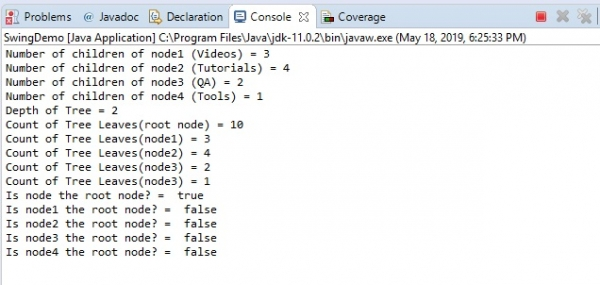# Check whether a node is a root node or not in JTree

To check whether a node is a root node or not, use the isRoot() method. This returns a boolean value. TRUE if the node is a root node, else FALSE is returned. For example, TRUE is returned since the following node is a root node −

node.isRoot()

Another example, FALSE is returned since the following node isn’t a root node −

node2.isRoot()

The following is an example to check whether a node is a root node or not −

## Example

package my;
import javax.swing.JFrame;
import javax.swing.JTree;
import javax.swing.tree.DefaultMutableTreeNode;
public class SwingDemo {
public static void main(String[] args) throws Exception {
JFrame frame = new JFrame("Demo");
DefaultMutableTreeNode node = new DefaultMutableTreeNode("Website");
DefaultMutableTreeNode node1 = new DefaultMutableTreeNode("Videos");
DefaultMutableTreeNode node2 = new DefaultMutableTreeNode("Tutorials");
DefaultMutableTreeNode node3 = new DefaultMutableTreeNode("QA");
DefaultMutableTreeNode node4 = new DefaultMutableTreeNode("Tools");
DefaultMutableTreeNode one = new DefaultMutableTreeNode("PHP Videos");
DefaultMutableTreeNode two = new DefaultMutableTreeNode("HTML5 Videos");
DefaultMutableTreeNode three = new DefaultMutableTreeNode("BlockchainVideos");
DefaultMutableTreeNode four = new DefaultMutableTreeNode("Java");
DefaultMutableTreeNode five = new DefaultMutableTreeNode("DBMS");
DefaultMutableTreeNode six = new DefaultMutableTreeNode("CSS");
DefaultMutableTreeNode seven = new DefaultMutableTreeNode("MongoDB");
DefaultMutableTreeNode eight = new DefaultMutableTreeNode("Python QA");
DefaultMutableTreeNode nine = new DefaultMutableTreeNode("jQuery QA");
DefaultMutableTreeNode ten = new DefaultMutableTreeNode("Photo Editing Tool");
JTree tree = new JTree(node);
for (int i = 0; i < tree.getRowCount(); i++) {
tree.expandRow(i);
}
tree.putClientProperty("JTree.lineStyle", "Angled");
System.out.println("Number of children of node1 (Videos) = " +node1.getChildCount());
System.out.println("Number of children of node2 (Tutorials) = " +node2.getChildCount());
System.out.println("Number of children of node3 (QA) = " +node3.getChildCount());
System.out.println("Number of children of node4 (Tools) = " +node4.getChildCount());
System.out.println("Depth of Tree = " + node.getDepth());
System.out.println("Count of Tree Leaves(root node) = " +node.getLeafCount());
System.out.println("Count of Tree Leaves(node1) = " +node1.getLeafCount());
System.out.println("Count of Tree Leaves(node2) = " +node2.getLeafCount());
System.out.println("Count of Tree Leaves(node3) = " +node3.getLeafCount());
System.out.println("Count of Tree Leaves(node3) = " +node4.getLeafCount());
System.out.println("Is node the root node? = " + node.isRoot());
System.out.println("Is node1 the root node? = " + node1.isRoot());
System.out.println("Is node2 the root node? = " + node2.isRoot());
System.out.println("Is node3 the root node? = " + node3.isRoot());
System.out.println("Is node4 the root node? = " + node4.isRoot());
tree.setRowHeight(25);
frame.setSize(600,450);
frame.setVisible(true);
}
}

## OutputFollowing is our JTree −